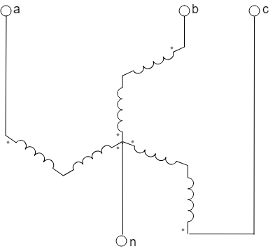# Earthing Transformer

Three-phase earthing transformer in zigzag configuration

• Library:
• Simscape / Electrical / Passive / Transformers

•## Description

The Earthing Transformer block models a three-phase zigzag-connection earthing or grounding transformer. The block allows you to provide an artificial neutral in an ungrounded three-phase power system. The artificial neutral creates a low-impedance path for the zero-sequence current and high-impedance path for the positive-sequence current. To accommodate a phase-to-neutral load, the return path allows a delta-connected supply.

For more information on using a three-phase zigzag-connection earthing or grounding transformer, see Engineering Applications### Equations

This block is implemented in both electrical and magnetic domains, representing the physics of phase windings and magnetic core. The equivalent circuit for the block includes windings, reluctances, and eddy currents.For a zigzag configuration in magnetic circuit, the a-. b-, and c-phases represent each leg of the magnetic core.

You can obtain the relationship between the electrical and the magnetic properties by applying several mathematical steps based on the magnetic flux conservation rule and on the Hopkinson's law.

For earthing transformers, the couplings between the phases and all the windings are identical. In the equations below:

• n is the number of turns of the winding.

• R is the magnetizing reluctance between phases.

• RL is the leakage reluctance.

• Leddy is the magnetic inductance due to eddy current losses between phases.

Then the relationship between the inductance matrix in electrical domain and the reluctance matrix in the magnetic domain can be written as:

`$L\text{​}=\left[\begin{array}{ccc}{L}_{11}& {L}_{12}& {L}_{13}\\ {L}_{21}& {L}_{22}& {L}_{23}\\ {L}_{31}& {L}_{32}& {L}_{33}\end{array}\right]=\left[\begin{array}{ccc}{n}^{2}\left(\frac{2}{Rl}+\frac{6}{R}\right)& -3\frac{{n}^{2}}{R}& -3\frac{{n}^{2}}{R}\\ -3\frac{{n}^{2}}{R}& {n}^{2}\left(\frac{2}{Rl}+\frac{6}{R}\right)& -3\frac{{n}^{2}}{R}\\ -3\frac{{n}^{2}}{R}& -3\frac{{n}^{2}}{R}& {n}^{2}\left(\frac{2}{Rl}+\frac{6}{R}\right)\end{array}\right]$`

The diagonal elements represent the sum of a leakage and magnetizing inductance for each phase, such that:

`${L}_{l}=\frac{2{n}^{2}}{Rl}$`

Therefore:

`${R}_{L}=\frac{2{n}^{2}}{{L}_{l}}$`

`$R=\frac{9{n}^{2}}{{L}_{m}}$`

`${L}_{eddy}=\frac{9{n}^{2}}{{R}_{m}}$`

## Ports

### Conserving

expand all

Expandable three-phase electrical conserving port associated with three-phase voltage.

Electrical three-phase electrical conserving port associated with the neutral point.

## Parameters

expand all

Whether to model composite or expanded three-phase ports.

Composite three-phase ports represent three individual electrical conserving ports with a single block port. You can use composite three-phase ports to build models that correspond to single-line diagrams of three-phase electrical systems.

Expanded three-phase ports represent the individual phases of a three-phase system using three separate electrical conserving ports.

Rated apparent power in SI units. The value must be greater than `0`.

Rated voltage in SI units. The value must be greater than 0.

Rated electrical frequency in SI units. The value must be greater than `0`.

System of units for impedance parameterization.

#### Dependencies

The visibility of other parameters depends on the value of this parameter.

Per-unit zero-sequence resistance. The value must be greater than `0`.

#### Dependencies

This parameter is only visible when the Units parameter is set to `Per unit`.

Per-unit zero-sequence reactance. The value must be greater than `0`.

#### Dependencies

This parameter is only visible when the Units parameter is set to `Per unit`.

Per-unit shunt magnetizing resistance. The value must be greater than `0`.

#### Dependencies

This parameter is only visible when the Units parameter is set to `Per unit`.

Per-unit shunt magnetizing reactance. The value must be greater than `0`.

#### Dependencies

This parameter is only visible when the Units parameter is set to `Per unit`.

Zero-sequence resistance in SI units. The value must be greater than `0`.

#### Dependencies

This parameter is only visible when the Units parameter is set to `SI`.

Zero-sequence inductance in SI units. The value must be greater than `0`.

#### Dependencies

This parameter is only visible when the Units parameter is set to `SI`.

Shunt magnetizing resistance in SI units. The value must be greater than `0`.

#### Dependencies

This parameter is only visible when the Units parameter is set to `SI`.

Shunt magnetizing inductance in SI units. The value must be greater than `0`.

#### Dependencies

This parameter is only visible when the Units parameter is set to `SI`.